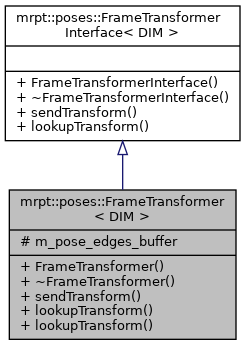MRPT  1.9.9
mrpt::poses::FrameTransformer< DIM > Class Template Referenceabstract

## Detailed Description

### template<int DIM> class mrpt::poses::FrameTransformer< DIM >

See docs in FrameTransformerInterface.

This class is an implementation for standalone (non ROS) applications.

FrameTransformerInterface

Definition at line 89 of file FrameTransformer.h.

`#include <mrpt/poses/FrameTransformer.h>`

Inheritance diagram for mrpt::poses::FrameTransformer< DIM >:## Classes

struct  TF_TreeEdge

## Public Types

using base_t = FrameTransformerInterface< DIM >

using pose_t = typename SE_traits< DIM >::pose_t
This will be mapped to CPose2D (DIM=2) or CPose3D (DIM=3) More...

using lightweight_pose_t = typename SE_traits< DIM >::lightweight_pose_t
This will be mapped to mrpt::math::TPose2D (DIM=2) or mrpt::math::TPose3D (DIM=3) More...

## Public Member Functions

FrameTransformer ()

~FrameTransformer ()

virtual void sendTransform (const std::string &parent_frame, const std::string &child_frame, const typename base_t::pose_t &child_wrt_parent, const mrpt::system::TTimeStamp &timestamp=mrpt::system::now()) override

virtual FrameLookUpStatus lookupTransform (const std::string &target_frame, const std::string &source_frame, typename base_t::lightweight_pose_t &child_wrt_parent, const mrpt::system::TTimeStamp query_time=INVALID_TIMESTAMP, const double timeout_secs=.0) override
Queries the current pose of `target_frame` wrt ("as seen from") `source_frame`. More...

FrameLookUpStatus lookupTransform (const std::string &target_frame, const std::string &source_frame, typename base_t::pose_t &child_wrt_parent, const mrpt::system::TTimeStamp query_time=INVALID_TIMESTAMP, const double timeout_secs=.0)

virtual void sendTransform (const std::string &parent_frame, const std::string &child_frame, const pose_t &child_wrt_parent, const mrpt::system::TTimeStamp &timestamp=mrpt::system::now())=0
Publish a time-stampped transform between two frames. More...

## Protected Types

using pose_tree_t = mrpt::aligned_std_map< std::string, mrpt::aligned_std_map< std::string, TF_TreeEdge > >

## Protected Attributes

pose_tree_t m_pose_edges_buffer

## ◆ base_t

template<int DIM>
 using mrpt::poses::FrameTransformer< DIM >::base_t = FrameTransformerInterface

Definition at line 92 of file FrameTransformer.h.

## ◆ lightweight_pose_t

template<int DIM>
 using mrpt::poses::FrameTransformerInterface< DIM >::lightweight_pose_t = typename SE_traits::lightweight_pose_t
inherited

This will be mapped to mrpt::math::TPose2D (DIM=2) or mrpt::math::TPose3D (DIM=3)

Definition at line 57 of file FrameTransformer.h.

## ◆ pose_t

template<int DIM>
 using mrpt::poses::FrameTransformerInterface< DIM >::pose_t = typename SE_traits::pose_t
inherited

This will be mapped to CPose2D (DIM=2) or CPose3D (DIM=3)

Definition at line 54 of file FrameTransformer.h.

## ◆ pose_tree_t

template<int DIM>
 protected

Definition at line 148 of file FrameTransformer.h.

## ◆ FrameTransformer()

template<int DIM>
 FrameTransformer::FrameTransformer ( )

Definition at line 41 of file FrameTransformer.cpp.

## ◆ ~FrameTransformer()

template<int DIM>
 FrameTransformer::~FrameTransformer ( )

Definition at line 45 of file FrameTransformer.cpp.

## ◆ lookupTransform() [1/2]

template<int DIM>
 FrameLookUpStatus FrameTransformer::lookupTransform ( const std::string & target_frame, const std::string & source_frame, typename base_t::lightweight_pose_t & child_wrt_parent, const mrpt::system::TTimeStamp query_time = `INVALID_TIMESTAMP`, const double timeout_secs = `.0` )
overridevirtual

Queries the current pose of `target_frame` wrt ("as seen from") `source_frame`.

It tries to return the pose at the given timepoint, unless it is INVALID_TIMESTAMP (default), which means returning the latest know transformation.

Definition at line 64 of file FrameTransformer.cpp.

Referenced by mrpt::poses::FrameTransformer< 2 >::lookupTransform(), and run_tf_test1().

## ◆ lookupTransform() [2/2]

template<int DIM>
 FrameLookUpStatus mrpt::poses::FrameTransformer< DIM >::lookupTransform ( const std::string & target_frame, const std::string & source_frame, typename base_t::pose_t & child_wrt_parent, const mrpt::system::TTimeStamp query_time = `INVALID_TIMESTAMP`, const double timeout_secs = `.0` )
inline

This is an overloaded member function, provided for convenience. It differs from the above function only in what argument(s) it accepts.

Parameters
 timeout_secs Timeout

Definition at line 111 of file FrameTransformer.h.

## ◆ sendTransform() [1/2]

template<int DIM>
 virtual void mrpt::poses::FrameTransformerInterface< DIM >::sendTransform ( const std::string & parent_frame, const std::string & child_frame, const pose_t & child_wrt_parent, const mrpt::system::TTimeStamp & timestamp = `mrpt::system::now()` )
pure virtualinherited

Publish a time-stampped transform between two frames.

## ◆ sendTransform() [2/2]

template<int DIM>
 void FrameTransformer::sendTransform ( const std::string & parent_frame, const std::string & child_frame, const typename base_t::pose_t & child_wrt_parent, const mrpt::system::TTimeStamp & timestamp = `mrpt::system::now()` )
overridevirtual

Definition at line 50 of file FrameTransformer.cpp.

Referenced by run_tf_test1().

## ◆ m_pose_edges_buffer

template<int DIM>
 pose_tree_t mrpt::poses::FrameTransformer< DIM >::m_pose_edges_buffer
protected

Definition at line 149 of file FrameTransformer.h.

 Page generated by Doxygen 1.8.14 for MRPT 1.9.9 Git: 7d5e6d718 Fri Aug 24 01:51:28 2018 +0200 at lun nov 2 08:35:50 CET 2020